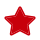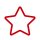Updated on 2023-03-14 GMT+08:00

# Cutting Strings

#### Function Name

\$strCut(String A,int B, int C)

#### Parameter Description

• String A: original string to be cut.
• int B: start subscript of the string to be cut, starting from 0.
• int C: end subscript of the string to be cut.

Parameters A, B, and C support the following types:

• Strings
• Environment parameters
• Local parameters

#### Function Description

Obtains the value string of a specified element and cuts it to a new string.

#### Application Scenarios

The string cutting function can be used in the following scenarios for API automation:

• Request URL
• Request body
• Checkpoint property
• if condition
• for loop interrupt condition

#### Example

• Request URL

As shown in the following figure, the value of test in the request URL is the string cutting function. Parameter A in the function is the environment parameter \$\${user}, parameter B is 2, and parameter C is 4.As shown in the following figure, the value of name in the request header is the string cutting function. Parameter A in the function is the environment parameter \$\${user}, parameter B is 2, and parameter C is 4.• Request body

As shown in the following figure, the request body uses the string cutting function. Parameter A in the function is the environment parameter \$\${user}, parameter B is 2, and parameter C is 4.• Checkpoint property

As shown in the following figure, the target value of the checkpoint property result is the string cutting function. Parameter A in the function is the environment parameter \$\${info}, parameter B is 2, and parameter C is 5.• if condition

As shown in the following figure, the target value of the if condition is the string cutting function. Parameter A in the function is abcdef, parameter B is 2, and parameter C is 4.• for loop interrupt condition

As shown in the following figure, the target value of the for loop interruption condition is the string cutting function. Parameter A in the function is the environment parameter \$\${test}, parameter B is 2, and parameter C is 4.